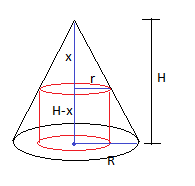# A right circular cylinder is placed inside a cone of radius R and height H so that the base of...

## Question:

A right circular cylinder is placed inside a cone of radius R and height H so that the base of the cylinder lies on the base of the cone.

a. Find the dimensions of the cylinder with maximum volume. Specifically, show that the volume of the maximum-volume cylinder is four ninths the volume of the cone.

b. Find the dimensions of the cylinder with maximum lateral surface area (area of the curved surface).

## Maximizing Volume and Surface Area:

The volume of a cylinder is equivalent to the area of its base times its height. The volume of a cone, on the other hand, is equivalent to one-third of the area of its base times its height. The lateral surface area of a cylinder is equivalent to the circumference of its circular base times its height. To maximize the volume or surface area of a cylinder, take its first derivative and equate it to 0.Let {eq}x {/eq} be the height of the smaller cone.

Then {eq}H-x {/eq} is the height of the cylinder.

Let {eq}r {/eq} be the radius of the...

Become a Study.com member to unlock this answer! Create your account